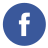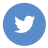### bitwise operation

programming

Bitwise operations are operations that are performed on the binary representation of integers. They allow you to manipulate individual bits within a number, and can be used to perform tasks such as setting, clearing, or toggling individual bits, or to check whether a particular bit is set or not.

There are several different bitwise operations:

• AND (&): This operation compares each bit of the first operand to the corresponding bit of the second operand, and if both bits are 1, the corresponding result bit is set to 1. Otherwise, the corresponding result bit is set to 0.

• OR (|): This operation compares each bit of the first operand to the corresponding bit of the second operand, and if either bit is 1, the corresponding result bit is set to 1. Otherwise, the corresponding result bit is set to 0.

• XOR (^): This operation compares each bit of the first operand to the corresponding bit of the second operand, and if the bits are different, the corresponding result bit is set to 1. Otherwise, the corresponding result bit is set to 0.

• NOT (~): This operation takes a single operand and inverts all of its bits (i.e., it changes 1s to 0s and 0s to 1s).

• LEFT SHIFT (<<): This operation shifts the bits of the first operand to the left by the number of positions specified by the second operand. This has the effect of multiplying the first operand by 2 to the power of the second operand.

• RIGHT SHIFT (>>): This operation shifts the bits of the first operand to the right by the number of positions specified by the second operand. This has the effect of dividing the first operand by 2 to the power of the second operand.

Here are some examples of bitwise operations in action:

``````# AND example
5 & 3  # Output: 1 (decimal)
# In binary:
#   5 is 101
#   3 is 011
#   Result is 001

# OR example
5 | 3  # Output: 7 (decimal)
# In binary:
#   5 is 101
#   3 is 011
#   Result is 111

# XOR example
5 ^ 3  # Output: 6 (decimal)
# In binary:
#   5 is 101
#   3 is 011
#   Result is 110

# NOT example
~5  # Output: -6 (decimal)
# In binary:
#   5 is 00000101
#   Result is 11111010

# LEFT SHIFT example
5 << 2  # Output: 20 (decimal)
# In binary:
#   5 is 101
#   Result is 10100

# RIGHT SHIFT example
5 >> 2  # Output: 1 (decimal)
# In binary:
#   5 is 101
#   Result is 001``````